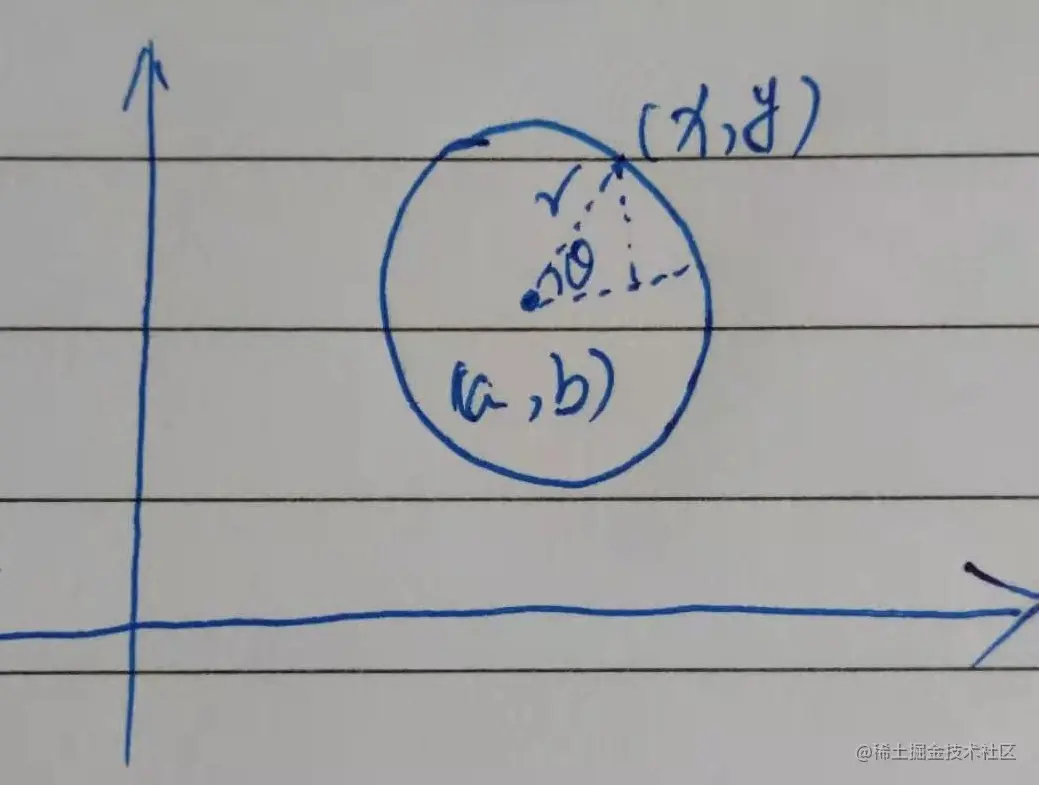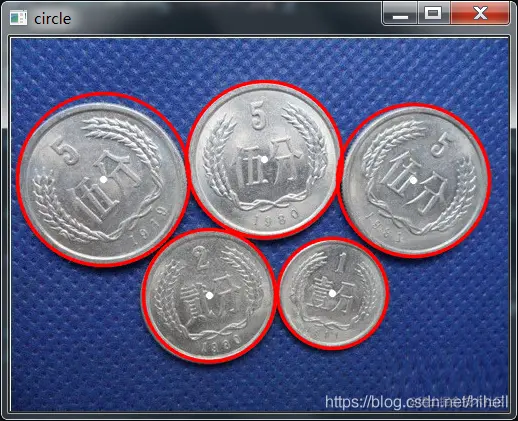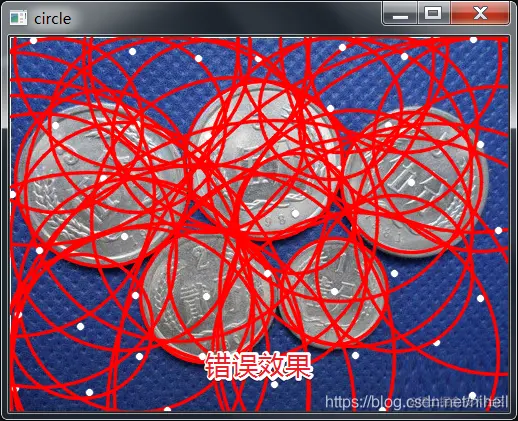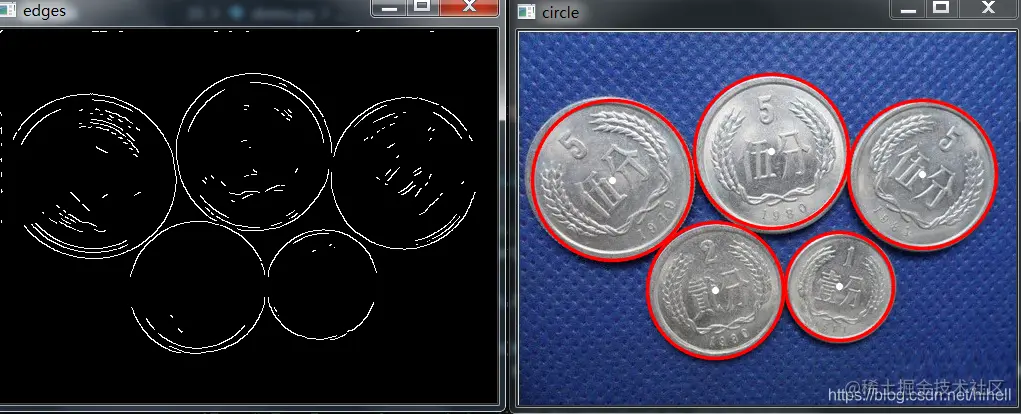# Application of Hoff circle detection in Python opencv

2022-01-30 00:48:47

This article has participated in  「 Digging force Star Program 」 , Win a creative gift bag , Challenge creation incentive fund .

Python OpenCV 365 Day study plan , Go into the field of image with the eraser . This blog is the third in this series 35 piece .

## Basic knowledge

By searching relevant information , Learned a little superficial knowledge of Hoff circle detection , Its basic content is that any non-zero pixel on the image , Can be a point on a potential circle . By voting , Generate cumulative coordinate plane , Then set a cumulative weight , De positioning circle .

In Cartesian coordinates, the equation of a circle is $(x-a)^2 + (y - a)^2=r^2$, among (a,b) It's the center of the circle ,r It's the radius , You can draw a picture to show .And according to the picture , We can see the following results

$x=a+r \cos \theta$ $y=b+ r\sin \theta$ That is, the following expression ： $a=x-r \cos \theta$ $b=y-r \sin \theta$

The next few sentences are not easy to understand , But there are a few conclusions that probably understand the meaning .

All circles passing through a point in Cartesian coordinate system , Mapping to abr The coordinate system is a three-dimensional curve Or explain it as , For Descartes xy A point in the plane $x_0,y_0$, Corresponding to abr Form a three-dimensional space , Is a space surface , about abr A point in the plane , Corresponding to Descartes xy Plane, it's a circle .

All circles passing through all non-zero pixels in Cartesian coordinate system , Constitute the abr There are many three-dimensional curves in the coordinate system In Cartesian coordinate system, all point equations on the same circle are the same , They map to abr The same point in the coordinate system , So when this point is accumulated to a certain amount （ Generally, it is set to be greater than a threshold ）, It can be regarded as a circle .

If in xy Three points on the plane $(x_0,y_0),(x_1,y_1),(x_2,y_2)$, stay abr Three dimensional space is the corresponding three spatial surfaces ( here abr It's a constant ）. The above content is described as the following equation ： $(x_0-a)^2 + (y_0 - a)^2=r^2$ $(x_1-a)^2 + (y_1 - a)^2=r^2$ $(x_2-a)^2 + (y_2 - a)^2=r^2$

Solve these three equations , We can get abr Value . This explanation $(x_0,y_0),(x_1,y_1),(x_2,y_2)$ These three points are determined by abr On the determined circle （ namely abr They represent the coordinates of the center of a circle (x,y) And the radius of the circle r）.

The above description is the principle of Standard Hough circle transformation , But the amount of calculation in three-dimensional space is very large , It is difficult to apply the Standard Hough circle variation to practice . Therefore, the functions involved in this paper , Hoff gradient method , Also called 2-1 Hough transform (21HT).

### The function prototype

python OpenCV Provides HoughCircles Function to find a circle , The function prototype is as follows ：

circles = cv2.HoughCircles(image, method, dp, minDist[, circles[, param1[, param2[, minRadius[, maxRadius]]]]])
Copy code 

The parameters are as follows ：

• image： The input image ;
• method： Method of detecting circle , At present, we support cv2.HOUGH_GRADIENT;
• dp： The accumulator resolution is inversely proportional to the image resolution ,dp Get bigger , The smaller the accumulator array , The general default is 1;
• minDist： The distance between the center of a circle ;
• param1： Edge detection gradient value ,Canny High threshold of function , Default 100;
• param2cv2.HOUGH_GRADIENT Accumulator threshold , The smaller the threshold , The more circles detected , Default 100;
• minRadius： Minimum radius , In pixels ;
• maxRadius： Maximum radius , In pixels ;

The parameters of each vector in the return value are ： The first element is the abscissa of the circle , The second is the ordinate , The third is the radius size .

Be careful ：minRadius and maxRadius You can better choose a circle , If you don't need to , Keep the default 0 that will do .

The test code is as follows , You can directly view the effect when running .

import cv2

#  Image preprocessing
gray = cv2.cvtColor(src, cv2.COLOR_BGR2GRAY)
img = cv2.medianBlur(gray, 7)

# TypeError: Argument 'radius' is required to be an integer
for x, y, r in circles:
cv2.circle(src, (int(x), int(y)), int(r), (0, 0, 255), 2, cv2.LINE_AA)
cv2.imshow('circle', src)
cv2.waitKey(0)

cv2.destroyWindow()
Copy code 

The operation effect is shown in the figure below , Although there is no problem with the test , But it takes a lot of time to adjust parameters , Mainly in the minDist Parameters 、param1 Parameter , If not adjusted slightly , The following picture will appear .Hoff circle detection is sensitive to noise , All Hough circle detection should be conducted with median filtering first . Denoising using Gaussian filter , Modify the code as follows ：

gaussian = cv2.GaussianBlur(gray, (7, 7),0)

Copy code 

If you want to add edge detection , Code tuning is even more cumbersome , For example, increase Canny operator

gaussian = cv2.GaussianBlur(gray, (7, 7),0)
#  utilize  Canny  Edge detection
edges = cv2.Canny(gaussian,160,180, apertureSize=3)
cv2.imshow("edges",edges)
Copy code 

The final effect is also satisfactoryIf the following error occurs during code running , Indicates that no circles were found , Continue to modify parameters .

TypeError: 'NoneType' object is not subscriptable
Copy code